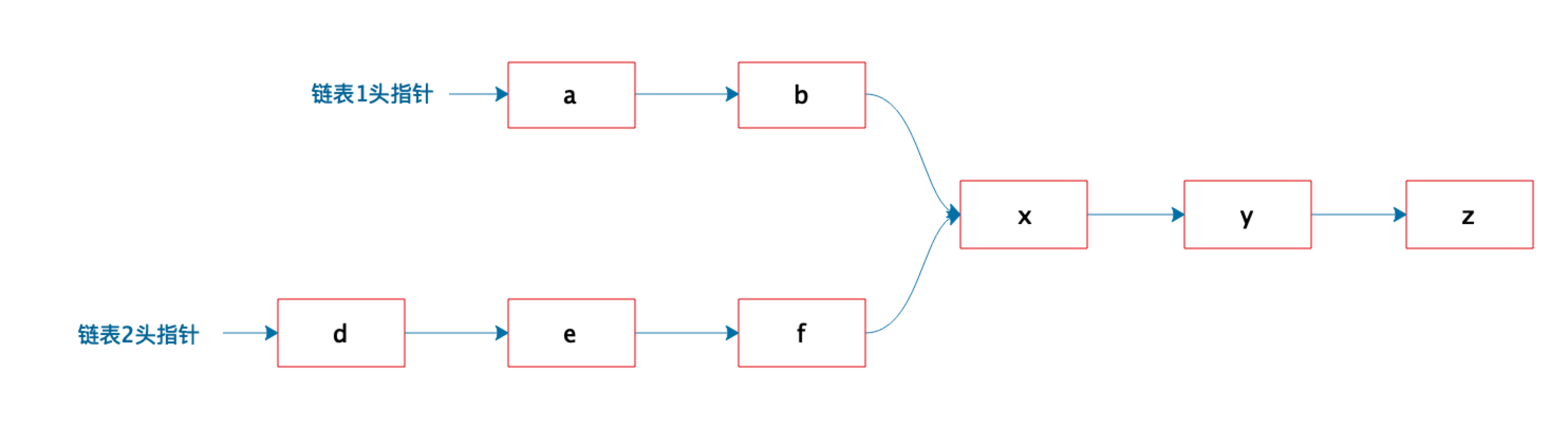# 架构师训练营第 8 周作业Season

## 题目

﻿﻿

## 题解1：直接法

Node getNode(Node h1, Node h2) {  Node next = h1;  HashSet set = new HashSet();  do {    if (next != null) {      set.add(next);    }    next = next.next();  } while(next != null);    for (Node n = h2; n != null; n = h2.next) {    if (set.contains(n)) {      return n;    }  }  return null;}

﻿

## 题解2： 双指针法

Node getNode(Node h1, Node h2) {  Node n1 = h1;  Node n2 = h2;    while (true) {    if (n1 == n2) {      return n1;    }    n1 = n1.next() == null ? h1 : n1.next();    n2 = n2.next() == null ? h2 : n2.next();  }}

﻿## 评论